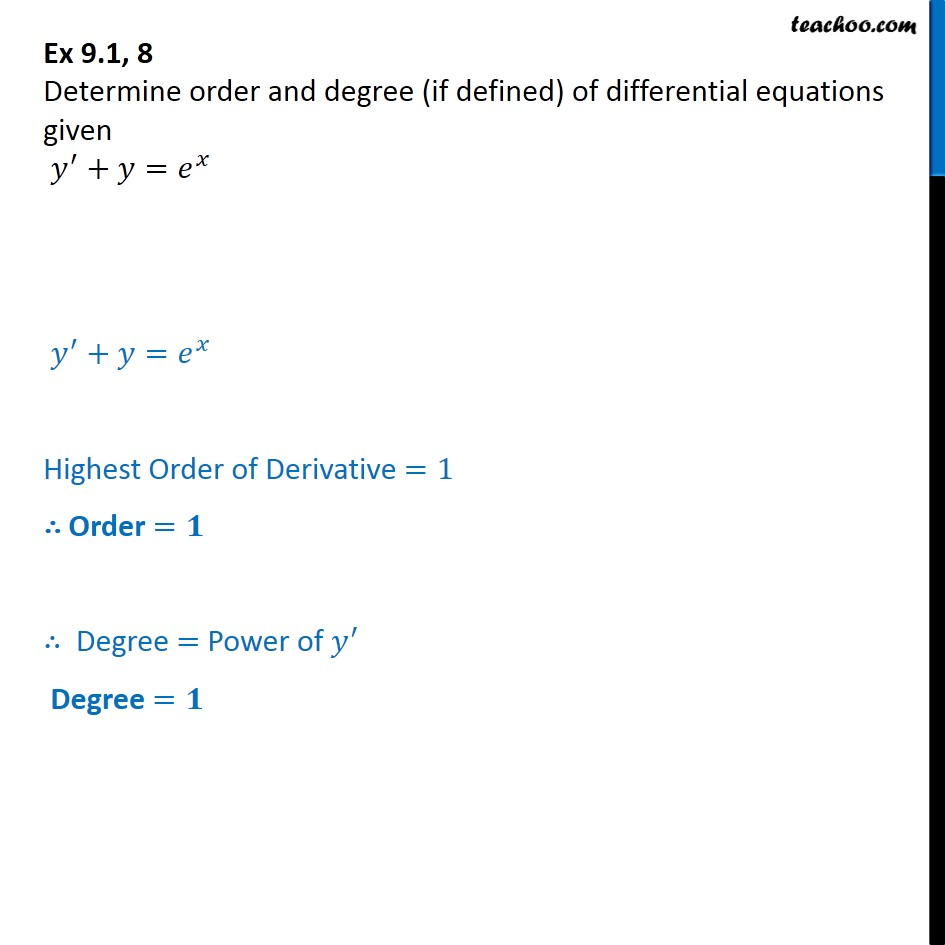Ex 9.1

Chapter 9 Class 12 Differential Equations (Term 2)
Serial order wise### Transcript

Ex 9.1, 8 Chapter 9 Class 12 Differential Equations NCERT Book Determine order and degree (if defined) of differential equations given y' + y = e^x y' + y = e^x Highest Order of Derivative =1 Order = 1 Degree = Power of y' Degree = 1